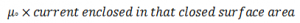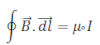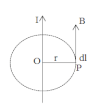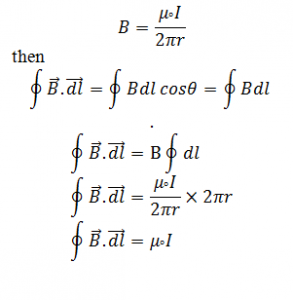## TyroCity

Physics XII Notes for Physics Notes

Posted on • Updated on

# Ampere’s Law

Ampere’s law is equivalent to the Gause law in electrostatics which measures the tangential component of magnetic field over any closed surface. Ampere’s law is alternative method of Biot and Savart law to measure magnetic field due to current carrying conductor.

Ampele’s Law can be defined as the line integral of magnetic field due to any close surface area is equal to:Mathematically,Proof of Ampere’s lawConsider a straight conductor carrying current I is as shown in figure. Let P be the point at a distance r from conductor where we have to draw a concentric circle of radius r. The direction of magnetic field at pint P is given by the tangent of the circle. Where the direction of B and dl is along the same direction and angle between them is 0°. We know the magnetic field due to straight current carrying conductor is: